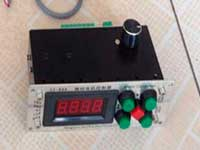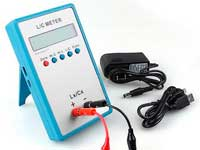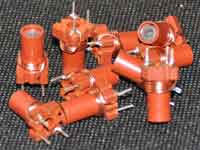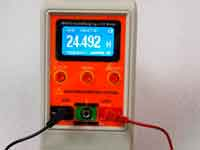Rating 0.00 (0 Votes)

Agreement on the dimensions and notations

Different programs (or different versions of this software)  are using different methods of measurement and notation of coil sizes. In order to avoid confusion and mistakes on this page details the nuances of this issue.

First of all, it concerns a single-layer coil. Numerical methods of the inductance calculation are based on on the assumption that the coil is wound by infinitely thin wire. The real thickness of the wire is taken into account in the future through adding corrections, however, the initial assumption leads to the fact that all dimensions in the original calculation formulas are measured from center to center of wire:• D - diameter of the winding
• l - the length of the winding
• p - the pitch of the winding
• d - wire diameter
• di - the diameter of the wire in the insulation

For all empirical formulas, this rule is retained. Often in articles on this site, it is possible to meet the concept of the form-factor of the coil. This is the ratio of the length to the diameter of the winding is l/D. Coil with a large l/D ratio is called the solenoid.

To simplify measurements for designers, in the program Coil32 the coil-former dimensions and nominal diameter of the wire (no insulation!)  are measured and then on the basis of these measurements, the program calculates the required dimensions "from center to center", which are used in numerical algorithms and in empirical formulas. This applies to both single-layer and multilayer coils, as well as other types of inductors. All dimensions and notation are clear from the images in the program. As an example, for single-layer coils see figure below:• Dc - the diameter of the coil
• lc - the length of the coil
• p - the pitch of the winding
• d - nominal diameter of the wire
• di - the diameter of the wire in the insulation

Thus, if we are talking about the size of a coil-former upon which the coil is wound, we will use the notion of the coil diameter and the coil length, if we are talking about the coil, we will use the concepts of the winding diameter and winding length, measured from center to center of wire.

It should be noted that because the insulation thickness is the parameter is not zero, even when winding a coil to a coil, the winding pitch p is always greater than the nominal wire diameter d. This is taken into account in the program.

Сomments from anonymous guests are enabled with moderation.

Coil Winding MachineUS \$187.53
The system consists of individual components including a controller, a stepping motor, a foot switch, power adapter and output shaft. This design makes it very easy for you to integrate the system into your own project.

LC Meter LC200AUS \$37.99
Measuring Capacitance Range:
0.01 pF - 100mF
Measuring Inductance Range:
0.001 uH - 100H
Measurement accuracy:1%.

RF InductorsUS \$6.43
Variable Ferrite Core Inductor coil set 3.5Turns 25-100MHZ

Auto Ranging LCR MeterUS \$63.07
Meter can measure 0.00pF to 100.00mF capacitance, 0.000uH to 100.00H inductance and 0.00? to 10.000M? resistance. Very suitable for small value capacitor and inductor measurement.